Electron. J. Diff. Equ., Vol. 2014 (2014), No. 263, pp. 1-13.

### Critical exponent and blow-up rate for the omega-diffusion equations on graphs with Dirichlet boundary conditions Weican Zhou, Miaomiao Chen, Wenjun Liu

Abstract:
In this article, we study the-diffusion equation on a graph with Dirichlet boundary conditions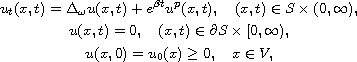where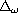is the discrete weighted Laplacian operator. First, we prove the existence and uniqueness of the local solution via Banach fixed point theorem. Then, by the method of supersolutions and subsolutions we prove that the-diffusion problem has a critical exponent: when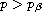, the solution becomes global; while when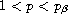, the solution blows up in finite time. Under appropriate hypotheses, we estimate the blow-up rate in the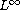-norm. Some numerical experiments illustrate our results.

Submitted July 10, 2014. Published December 22, 2014.
Math Subject Classifications: 35R02, 35B44, 35B51.
Key Words: Omega-diffusion equation; critical exponent; blow up; blow-up rate; graph.

Show me the PDF file (297 KB), TEX file, and other files for this article.Weican Zhou College of Mathematics and Statistics Nanjing University of Information Science and Technology Nanjing 210044, China email: 000496@nuist.edu.cn Miaomiao Chen College of Mathematics and Statistics Nanjing University of Information Science and Technology Nanjing 210044, China email: mmchennuist@163.com Wenjun Liu College of Mathematics and Statistics Nanjing University of Information Science and Technology Nanjing 210044, China email: wjliu@nuist.edu.cn